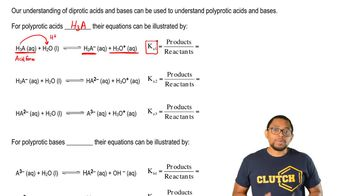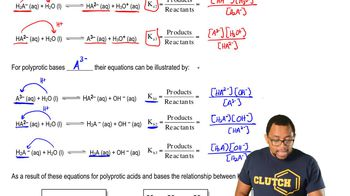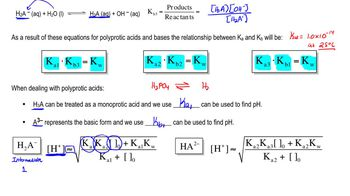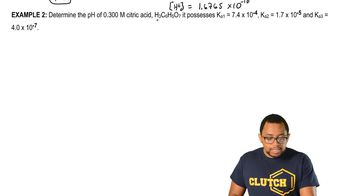Start typing, then use the up and down arrows to select an option from the list.# Polyprotic Acids

Jules Bruno
297views
1
So like we said, when it comes to polic product acids and bases, there's four different forms. There is the acidic form, the to intermediate forms and in the basic form. Now, when dealing with Polly product acids, we're gonna say here that the acidic form, which is H three a by default on, can be treated as a mantra. Protic acid and will use K A one than a three minus, which is the basic form can be treated as the model product base. So use K B one now based on issues with, um logs as well as concentrations. When it comes to calculating the pH off the to intermediate forms. Just keep in mind these two different types of equations that help us calculate H plus very quickly. And from there we can calculate pH. So let's take a look. So here this is the first intermediate form. So intermediate one, after it's lost its first acidic hydrogen, we're gonna say H plus is approximately equal to, so that's what that wavy line means you do. What you do here is you take the K one, you multiply it by K two times the initial concentration off your intermediate one form. So, for example, phosphoric acid represents a common try protic acid. So it takes away its first acidic hydrogen to give us the first intermediate. We lose another acidic hydrogen to give us our second intermediate, and then phosphate represents the basic form. So here, if they told us we had 10 Moeller n a h two p 04 this would be the first intermediate form again. Remember, you usually won't find it this way. B h that we lose gets replaced by a metal. Okay, so this is the common way of depicting it. The initial concentration would be this number here, the 0.10 Moeller, which would plug in plus K one times You're K W, which is still 1.0 times 10 to the negative 14 divided by K one plus again, the initial concentration. When you take the square root of all of that, that's gonna give you the concentration of H plus. And if you know the concentration of H plus, just remember, Ph equals negative log of H plus. Then, if you're dealing with a second intermediate intermediate, too, this is after it's lost two of its acidic hydrogen, so it shifts a little bit. So now we're dealing with K two and K three. If we go back up to this, why is this? We'll realize that when we're dealing with the first intermediate in the formula, it's in between K one and K two. So that's why they were part of the formula. Now we're dealing with the second intermediate form and its in between two and K three. That's why, in its formula to figure out a plus concentration, we're using K two and K three. So, for example, I gave you 15 Mueller and then in a to h P 04 Okay, so that's the second intermediate form plug in the initial concentration, which is this number. Then it's K two times k w, divided by K two plus initial concentration, which gives us pH on, which gives us hpe plus take the negative log of it to find pH. So again, when you're dealing with the acidic form before it's lost, any hydrogen is use K a one to solve for H plus, which then helps us figure out pH. If you're dealing with the form that has no acidic hydrogen, that's the basic form. So we deal with K B one for the intermediate forms. It's important to remember these two equations. If you're faced with questions like this MAWR, commonly, you're going to see the acidic form of the basic form these equations air good if you're approaching any types of homework, questions that ask you to figure out the pH of any one of the intermediate forms. So keep in mind the different approaches that come with calculating pH for the different types of Polly Protic acid forms.03:0104:1204:0805:1806:56